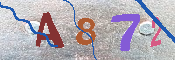## 生命起源与进化的几何图景——关于人和宇宙的设想（4）

1. 生命诞生前的准备

L是分子生命的线形，具有“可延伸的对称性”，

J是外界的直线作用，

G是外界有效作用区域，

2. 生命的起源与进化

a-b，

c-d，

…-x-y-z-w-…

…-y-x-w-z-…，

t(M)=>t(M-1)=>……=>t(0)，

L(2)=>

L(2)+L(t(M))=>

L(2)+L(t(M))+L(t(M-1))=>

……=>

L(2)+L(t(M))+……+L(t(1))=>

L(2)+L(t(M))+……+L(t(1))+L(t(0))，

L(2)+L(t(M))+……+L(t(1))+L(t(0))=L*